Summand

One of the summands is 145. The second is 10 more. Determine the sum of the summands.

Result

x =  300

Solution:Leave us a comment of example and its solution (i.e. if it is still somewhat unclear...):Be the first to comment!Next similar examples:

1. Three numbers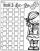How much we increases the sum of three numbers when the first enlarge by 14, second by 15 and third by 16? Choose any three two-digit numbers and prove results.
2. Tulips and daffodils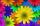Farm cultivated tulips and 211 units on 50 units more daffodils. How many spring flowers grown together?
3. What is missing (1000)What number is to add to get 1000?
4. Voting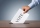400 employees cast their votes in a board member election that has only 2 candidates. 120 people vote for candidate A, while half of the remaining voters abstain. How many votes does candidate B receive?
5. Parenthesis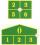Fill in the examples correctly brackets: A) 7.9+12:3-2 =23 B) 7.9+12:3-2=75
6. Roman numeralsWrite numbers written in Roman numerals as decimal.
7. Number unknownAdela thought the two-digit number, she added it to its ten times and got 407. What number does she think?
8. I thinkI think a number. When I multiply it by five, and after that I subtract 477, I get the same number as if I multiplied it twice. What number do I think?
9. Mystery numberA mystery number is between 800 and 920. No digits are the same. The difference of the number is 5. What is the mystery number?
10. Roman numerals 2+Add up the number writtens in Roman numerals. Write the results as a roman numbers.
11. Roman numerals +Add up the number writtens in Roman numerals. Write the results as a decimal number.
12. Multiples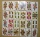What is the sum of the multiples of number 7 that are greater than 30 but less than 56?
13. Decimal expansion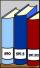Calculate: 2 . 1 + 0 . 10 + 7 . 10000 + 4 . 1000 + 6 . 100 + 0 . 100000 =
14. CollectionMajka gave from her collection of calendars Hanke 15 calendars, Julke 6 calendars and Petke 10 calendars. Still remains 77 calendars. How many calendars had Majka in her collection at the beginning?Add two mixed fractions: 2 4/6 + 1 3/6At the florist are 50 tulips and 5 times less roses. How many flowers are in flower shop?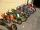The car park has 45 motorcycles and 25 cars. 14 cars went away and 2 arrived, then departs 12 motorcycles and 2 arrive. How many motorbikes and cars there are now?